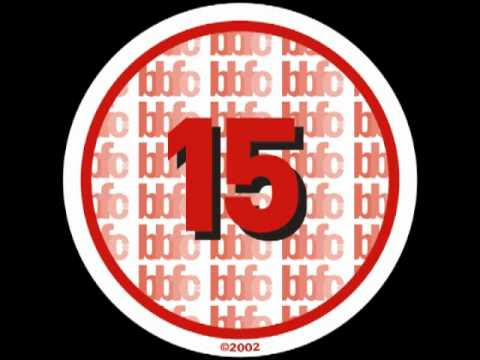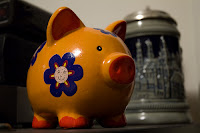## Posts

Showing posts from January, 2012

### Free ebook "All Children Can Be Great Listeners"... this free e-book is meant for preschool-kindergarten age kids, and you can download it here. It's not my book, but courtesy of  Renee from SchoolSparks.com

### Triangle problem with equal areas - solutionThis is the solution for the triangle problem with equal areas that I posted earlier.

We are given that the areas of the three right triangles are equal, that is the area of the triangle DAE = area of the triangle EBF = area of the triangle FCD.

We will make an equation based on that fact. For that, I like to use x as my variable, so I denote the longer side of the rectangle with a, the other side with b, the distance AE with x, and the distance BF with y. We are asked the ratio AE:EB, which is the same as x : (a − x) using my notation, and the ratio BF:FC, which is the same as y : (b − y) using my notation.

The area of triangle ADE is its base times altitude divided by 2, or bx/2.
The area of triangle EBF is its base times altitude divided by 2, or y(a − x)/2.
The area of triangle CDF is its base times altitude divided by 2, or a(b − y)/2.

And these three are equal. Basically you just make two equations from the above information, and manipulate your equations until you get the ratio…Bon from Math is not a four-letter word made this little counting song, sung to the tune of "Twinkle Twinkle Little Star". Hope your little ones enjoy it!

### Three-day sale for Math Mammoth

SORRY I forgot to post it here (I just sent this to my email list).

For January 23-25, get 23% off of all Math Mammoth downloads & CDs
at Kagi store.

Use the coupon code THREEDAYS.

Go to http://www.mathmammoth.com first, then find the links to
Kagi's order pages. OR, use these direct links:

~ Light Blue series (complete curriculum)
https://store.kagi.com/cgi-bin/store.cgi?storeID=5KN_LIVE&page=Math_Mammoth_LightBlue_Series

~ Blue series
https://store.kagi.com/cgi-bin/store.cgi?storeID=5KN_LIVE

~ Golden and Green Series
https://store.kagi.com/cgi-bin/store.cgi?storeID=5KN_LIVE&page=MathMammoth_Workbooks

~ Make It Real Learning workbooks
https://store.kagi.com/cgi-bin/store.cgi?storeID=5KN_LIVE&page=Make_It_Real_Learning

https://store.kagi.com/cgi-bin/store.cgi?storeID=5KN_LIVE&page=Math_Mammoth_Pack…

### Triangle puzzle - equal areasI hope Pat doesn't mind that I copied the image from his blog... He posted this triangle puzzle on his blog and I thought you might enjoy it, too!

Basically, we have a triangle DFE inside a rectangle, dividing the rectangle into various triangles.  And, the three areas of fainter color are equal. That is, the area of the triangle DAE = area of the triangle EBF = area of the triangle FCD. (Notice the image is not drawn to scale at all.)

And, we're asked to solve the RATIOS AE : EB and BF : FC.

The solution is here.

### Versa RulerWell, this IS something different! A physical ruler that can draw shapes with any angle measure you want.

Unfortunately it's not yet in production. In fact, the project is needing funding... but very interesting! Please read more at

Rule Like Never Before! NEW Shape-making Versa Ruler

This ruler give you accurate measurements for every side and angle. You  can connect sides to form angles, which scale and skew smoothly, and you can lock angles and sides.  Cool!

### Vi Hart and mathematical doodlingI just learned about Vi Hart's "doodling in math class" videos (hat tip goes to Fawn Nguyen).

Vi calls herself mathemusician - and definitely, she's a talented and smart gal! I'm sure you'll enjoy her videos (as long as you can follow her super fast speeaking). Here are some that I enjoyed:

Doodling in Math: Spirals, Fibonacci, and Being a Plant [1 of 3]

Binary Hand Dance was pretty cool too!

Her series of "mathematical doodling" videos have become somewhat of a viral success. Here's one more:

Doodling in Math Class: Infinity Elephants

### Math teachers are at play againDenise's done a beautiful job with the current Math Teachers at Play carnival number 46, lots to read and explore and see... head on over!

### Compound interestPhoto courtesy of Mooster Someone sent me in a question concerning compound interest...
Please send me the formula for compound interest and explain line by line.

p(1 + rate)3

What does the 1 stand for and must you add it to the rate of say 10%?

100(1+10)3 years = ???

Obviously I am looking for a basic course?

The formula that this person is using is correct... the formula for compound interest is

A = p(1 + r)t

but this formula doesn't give us the amount of interest -- it gives us the amount of money you would withdraw after t years. In the formula, p is the original principal, r is the interest rate, and t is the time in years.

However, we cannot put the interest rate in as he did. If r = 10%, then r = 0.1 must be used in this formula. In other words, FIRST convert your percentage into a decimal.

For example, if the principal is \$5000 and r = 10% = 0.1, then we get

A = \$5000 × 1.1t

The number 1 in the formula p(1 + r)t doesn't stand for anything by itself. It come…